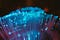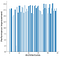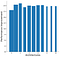# Autoencoder architecture optimization by genetic programming.Photo by Sander Weeteling on Unsplash

One of the main challenges in the development of neural networks is to determine the architecture. That means how the different layers are connected, the depth, the units in each layer, and the activation for each layer. In the following post, I will show a method to optimize the architecture of an autoencoder by genetic programming.

# Autoencoder

An autoencoder is a type of neural network that is trained to learn itself. The advantage of this kind of training is the generation of a lower-dimensional space that can represent the data. That means that an autoencoder can be used for dimensionality reduction. In this post we are going to use the MNIST data set to train an autoencoder with the following constrains, the first and last layer of the autoencoder will have the same size as the input, and the bottleneck representation will have two dimensions.

First, we are going to train a vanilla autoencoder with only three layers, the input layer, the output layer, and the bottleneck layer. The vanilla autoencoder will help us to select the best autoencoder generated the optimization strategies. To train the vanilla autoencoder we use the following, setting the training epochs to 25. The vanilla autoencoder can be also found in the Keras blog.

# Neural network generation

To dynamically generate a neural network first, we split the autoencoder into two functions one the encoder and the second model will be the decoder.

Each function will take an array of integers, the length of the array will be the depth of the network, and each integer will represent the number of dense units in the network.

Then a third function will work as a wrapper function, it will join the encoder and the decoder model and train the network. And will return the encoder model, to visualize the bottleneck representation and the full autoencoder network to evaluate the fitness of the network.

# Optimization strategies.

The optimization of the architecture of a neural network is an integer optimization problem, as all the parameters, depth and units are integers. Such problems can be solved by integer programming, or by a metaheuristic approach. Genetic algorithms can be classified as metaheuristics methods, we are going to use two methods: a single tournament search and a modified version of the differential evolution algorithm. A key advantage of genetic algorithms is the ability to handle hard problems, problems that don’t have a derivative, and the simplicity of the implementation in some cases. However, the biggest disadvantage is that genetic algorithms do not guarantee that an optimal solution is ever found.

Tournament search

In this method a population of possible solutions is generated, then the fitness of the population is measured. In this case, the fitness will be the loss at the last step of training. Then the individual with the best performance is selected as the solution to the problem.

Evolutionary approach

The algorithm starts by creating a population of candidate solutions, best solutions are selected based on its fitness. Then the selected solutions are mutated to generate new candidates solutions. Also, new randomly generated candidate solutions are added to the population. The algorithm finishes when the number of generations is reached.

To optimize the architecture of the neural network, both strategies will return a vector with the number of units in a dense layer, and the number of elements in that vector will be the depth of the network

# Optimization results

With the performance obtained from every iteration from each method, we can evaluate the performance of the models and the improvement in comparison with the vanilla autoencoder. On the plots, the height of the bar represents the percentage of improvement and the width represents the number of parameters.Tournament search optimization

With that visualization strategy, we can focus on selecting the best performance with the least number of parameters, or to select the best model and have an idea on the number of parameters needed for the model.Evolutionary optimization

Now you can apply a genetic algorithm to optimize the network architecture of a small variational autoencoder network, how to visualize the results, and compare several neural networks. And in the next post of this series, I will show you how to analyze and optimize the weights in a trained neural network.

Writing about math, natural sciences and academia.

## More from Octavio Gonzalez-Lugo

Writing about math, natural sciences and academia.